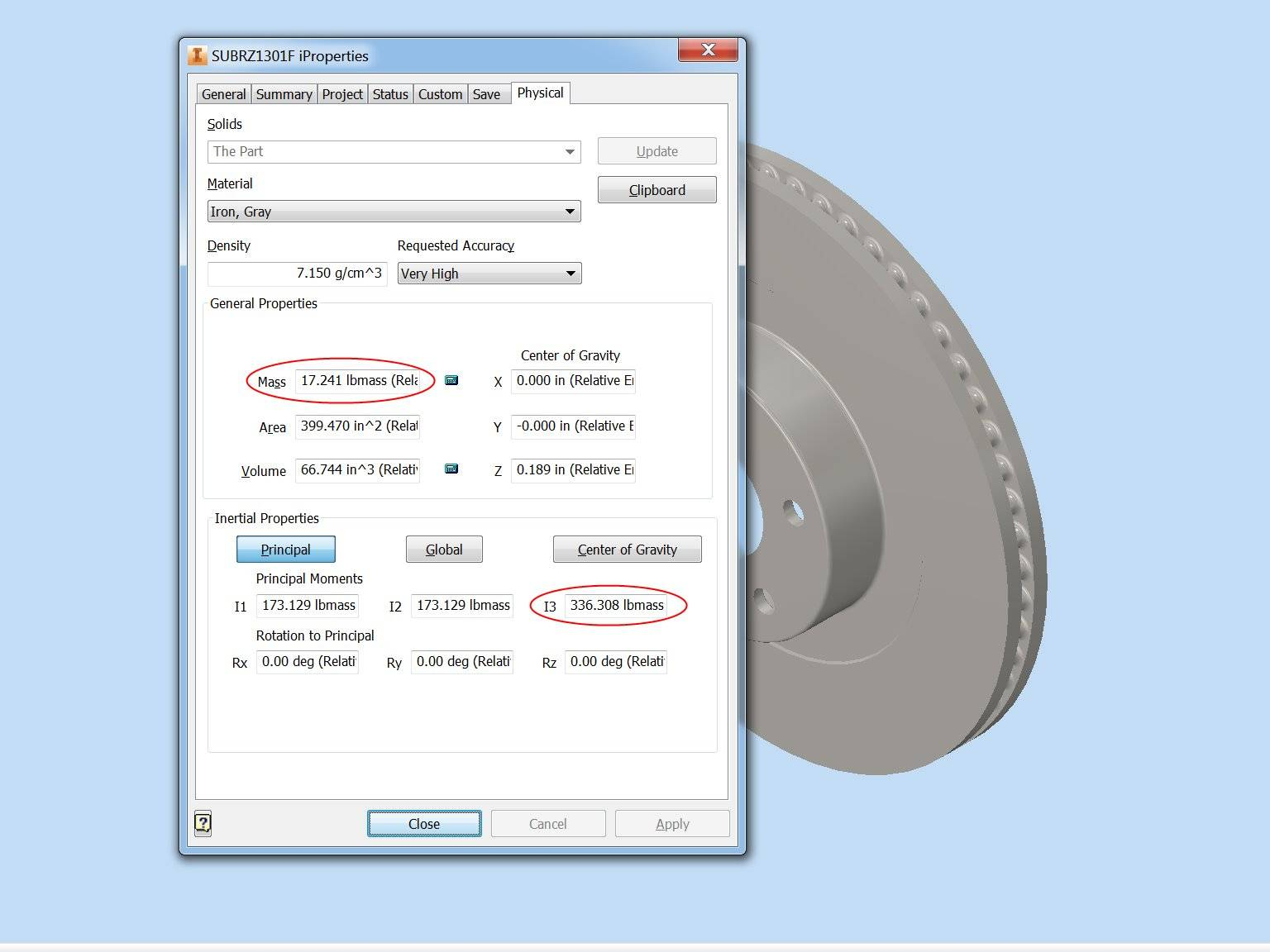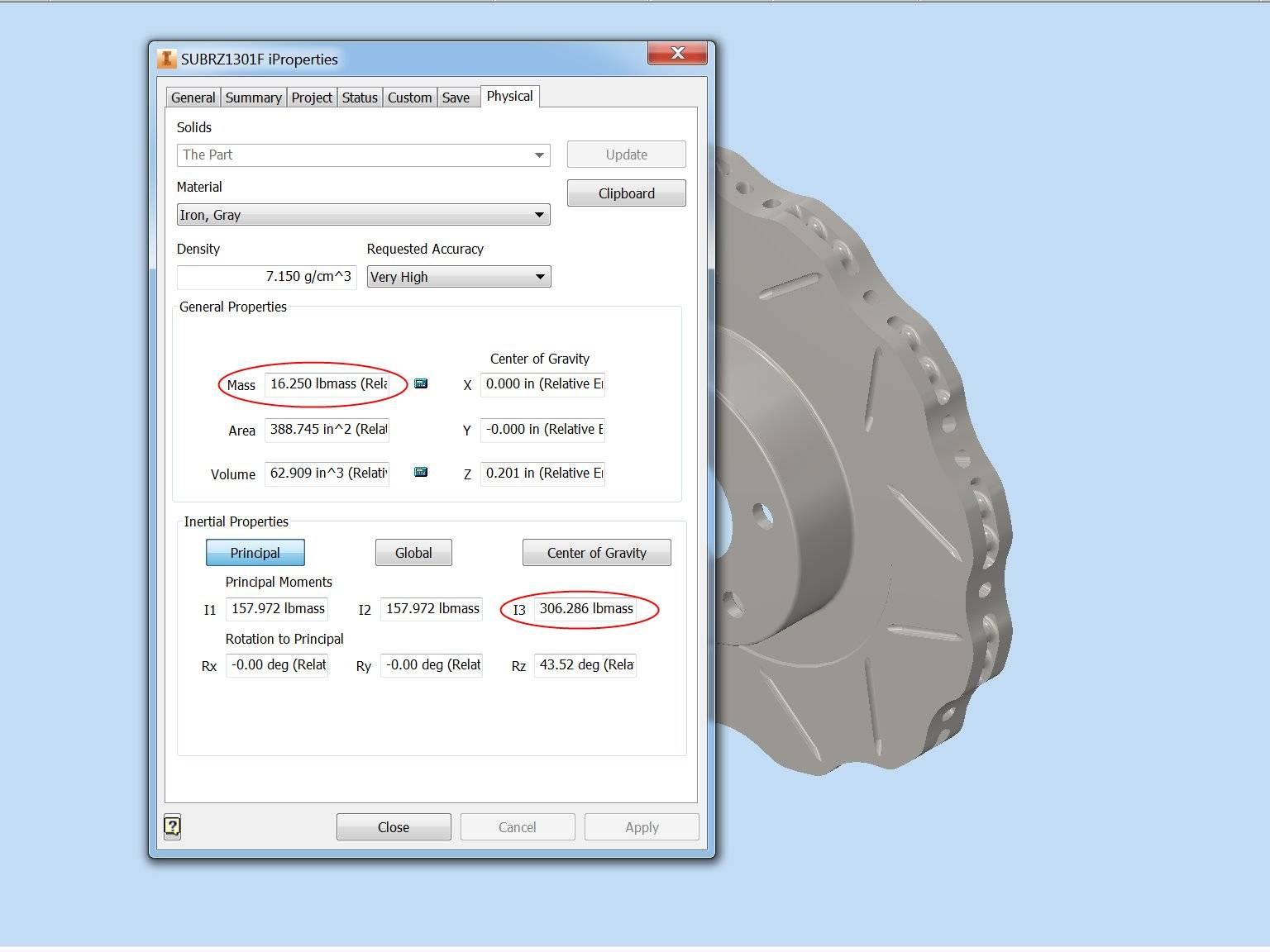# Technical Development & Design

#### Background

Drawing from successful brake technology in highly competitive motorcycle racing and combining the need for compact brakes for rally racing, the WaveSpec rotor was developed to increase braking performance while simultaneously decreasing rotating mass.

#### Rotating Mass

Our rotors reduce unsprung mass as well as moment of inertia. Commonly known as rotating mass, moment of inertia is the rotational equivalent of mass in Newton’s second law of motion. Most people are familiar with the linear version of Newton’s second law: F=ma, where Force equals Mass times Acceleration. In a rotating system, the formula is τ=Iα, where Torque equals Moment of Inertia times Angular Acceleration. Therefore, reducing the Moment of Inertia (MOI) is the angular equivalent of reducing mass.

The key to understanding MOI is that the farther away mass is distributed from the axis of rotation, the higher the moment of inertia is. MOI is calculated by multiplying the mass by the distance to the axis of rotation squared and is expressed by pound mass times inches squared (lbm*in^2). Since the distance component is squared, reducing mass at the outer edge of a rotating object such as a brake rotor has a much more significant impact on angular acceleration than reducing mass at the center of rotation.

On something like a brake rotor, calculating the MOI by hand is tedious since the mass distribution is not uniform. However, with modern Computer Aided Drafting and 3D modeling, the MOI can be calculated in tenths of a second. While any reduction of unsprung mass is highly desirable, the Wavespec design has an even more significant impact on MOI which directly translates to shorter stopping distances and improved acceleration.stock rotor (click to zoom)WaveSpec rotor (click to zoom)

#### Surface Area

The Wavespec design increases the surface area to volume ratio which means that for the thermal mass, there is more surface area to transfer heat to the surrounding air. Surface area to volume ratio is calculated by dividing the surface area by the volume. In a sample physical analysis, we compared the surface area to volume ratio on an OEM design of 5.985/in with the Wavespec design, and the Wavespec design has a ration of 6.179/in which is a 3.2% increase.